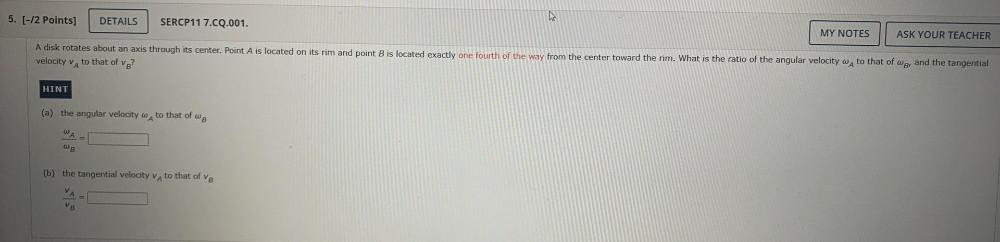Question:

# A disk rotates about an axis through its center. Point A is located on its rim and point B is located exactly one fourth of the way from the center toward the rim. What is the ratio of the angular velA disk rotates about an axis through its center. Point A is located on its rim and point B is located exactly one fourth of the way from the center toward the rim. What is the ratio of the angular velocity w A to that of wg, and the tangential velocity VA to that of velocity VB? (a) the angular velocity ωA to that of ωB ωA/ωB= (b) the tangential velocity VA to that of VB VA/VB=